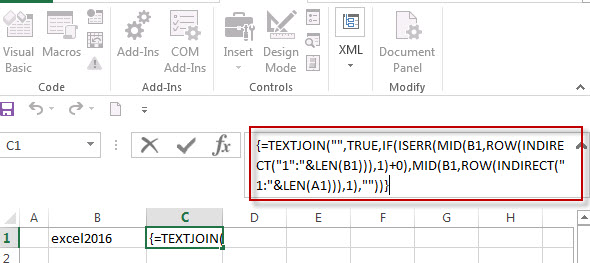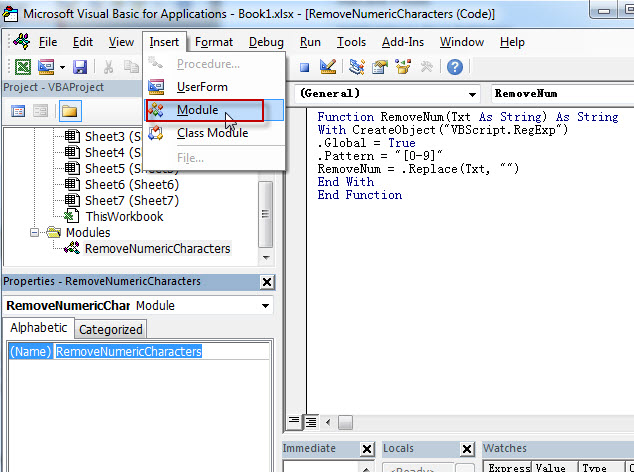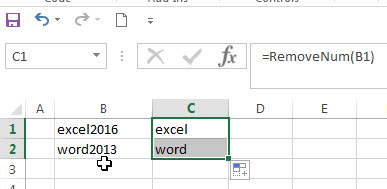# How to Remove Numeric Characters from a Cell

This post explains that how to remove numeric characters (numbers) from a text string in one cell in excel 2016. how to remove only numeric characters but keep the non-numeric characters from a cell containing text string with an excel formula. And how to remove numbers from a cell with a user defined function in excel VBA.

## Remove numeric characters from a cell containing text

If you want to remove numeric characters from alphanumeric string, you can use the following complex array formula using a combination of the TEXTJOIN function, the MID function, the Row function, and the INDIRECT function.

`{=TEXTJOIN("",TRUE,IF(ISERR(MID(B1,ROW(INDIRECT("1":"&LEN(B1))),1)+0),MID(B1,ROW(INDIRECT("1:"&LEN(A1))),1),""))}`

Let’s see how the above array formula works:

The MID function will extract the sub strings based on the start_num and num_chars arguments. And the INDIRECT function within ROW function will returns an array list, like below:

{1,2,3…} and the length of array is the length of CELL B1.

The MID function will use the result returned by the ROW function to extract the sub string from Cell B1, as the results by the ROW function is an array, so the MID function also returns an array list. Like below format:

`{"e","x","c","e","l","2","0","1","6}`

The result returned by MID function add zero for each value in array, if the value is non-numeric value, then the ISERR function return a #VALUE error. Or return the numeric text values will be converted to number.

the IF function will check if the result returned by ISERR function, If TRUE, then return an empty string, otherwise, return that non-numeric character using the second MID function.

`{"e","x","c","e","l","","","",""}`

Last, the TEXTJOIN function will join all values and ignore the empty string.## Remove numeric characters from text using a user defined function in VBA

You can create a new function to remove numeric characters from a cell that contain text string in Excel VBA. Just refer to the below steps:

1# open visual Basic Editor, then insert a module and name as : RemoveNumericCharacters.

2# click “Insert“->”Module“, then paste the following VBA code into the window:```Function RemoveNum(Txt As String) As String
With CreateObject("VBScript.RegExp")
.Global = True
.Pattern = "[0-9]"
RemoveNum = .Replace(Txt, "")
End With
End Function```

3# save the user defined function.

4# enter into the below formula in Cell C1.

`=RemoveNum(B1)`### Related Formulas

• remove non numeric characters from a cell
If you want to remove non numeric characters from a text cell in excel, you can use the array formula:{=TEXTJOIN(“”,TRUE,IFERROR(MID(B1,ROW(INDIRECT(“1:”&LEN(B1))),1)+0,””))}…
• Combine Text from Two or More Cells into One Cell
If you want to combine text from multiple cells into one cell and you can use the Ampersand (&) symbol.If you are using the excel 2016, then you can use a new function TEXTJOIN function to combine text from multiple cells…

### Related Functions

• Excel TEXTJOIN function
The Excel TEXTJOIN function joins two or more text strings together and separated by a delimiter. you can select an entire range of cell references to be combined in excel 2016.The syntax of the TEXTJOIN function is as below:= TEXTJOIN  (delimiter, ignore_empty,text1,[text2])…
• Excel MID function
The Excel MID function returns a substring from a text string at the position that you specify.The syntax of the MID function is as below:= MID (text, start_num, num_chars)…
• Excel LEN function
The Excel LEN function returns the length of a text string (the number of characters in a text string). The LEN function is a build-in function in Microsoft Excel and it is categorized as a Text Function.The syntax of the LEN function is as below:= LEN(text)…
• Excel IF function
The Excel IF function perform a logical test to return one value if the condition is TRUE and return another value if the condition is FALSE.The syntax of the IF function is as below:= IF (condition, [true_value], [false_value])….
• Excel ROW function
The Excel ROW function returns the row number of a cell reference.The ROW function is a build-in function in Microsoft Excel and it is categorized as a Lookup and Reference Function.The syntax of the ROW function is as below:= ROW ([reference])….
• Excel INDIRECT  function
The Excel ROW function returns the row number of a cell reference.The ROW function is a build-in function in Microsoft Excel and it is categorized as a Lookup and Reference Function.The syntax of the ROW function is as below:= ROW ([reference])….
• Excel ISERR function
The Excel ISERR function returns TRUE if the value is any error value except #N/A.The syntax of the ISERR function is as below:= ISERR (value)…